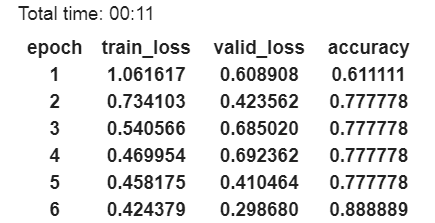# Checking that the results are not random

I am training a small dataset (15-20) images in the validation set, ~70 in the training set, and would like to verify that the results I get are not merely by chance.

I am interested in suggestions how best to do it.
Here is my take at it :

This is a sample run on the dataset.I have noticed that a) All runs were more successful than 60% b) the highest was around 88%
So, I have calculated 1) The probability of six experiments being all successful above 60 percent
2) The probability of having at least one experiment more successful than 85%.

Since the probability for each of those is bellow 0.05, I conclude that the results are statistically significant.

Is this a valid way of deciding? Are there better ones?